This page hosts a presentation / quick intro to tidyfun – enjoy!

## Example usage

The code in this page is drawn from tidyfun vignettes, and is intended as a quick introduction; for more details, please read the complete documentation!

If you haven’t installed tidyfun the code below will do so.

devtools::install_github("tidyfun/tidyfun")

Next I’ll load the package, as well as the tidyverse.

library(tidyfun)
## Warning: replacing previous import 'dplyr::data_frame' by 'vctrs::data_frame'
## Registered S3 method overwritten by 'GGally':
##   method from
##   +.gg   ggplot2
##
## Attaching package: 'tidyfun'
## The following objects are masked from 'package:stats':
##
##     sd, var
library(refundr)
##
## Attaching package: 'refundr'
## The following objects are masked from 'package:tidyfun':
##
##     chf_df, dti_df
library(tidyverse)

### Illustrative datasets

The datasets used in this vignette are the tidyfun::chf_df and tidyfun::dti_df dataset. The first contains minute-by-minute observations of log activity counts (stored as a tfd vector called activity) over seven days for each of 47 subjects with congestive heart failure. In addition to id and activity, we observe several covariates.

data(chf_df)

A quick plot of these data is below.

chf_df %>%
ggplot(aes(y = activity)) +
geom_spaghetti(alpha = .05)
## Warning: Unknown or uninitialised column: flipped_aes.

## Warning: Unknown or uninitialised column: flipped_aes.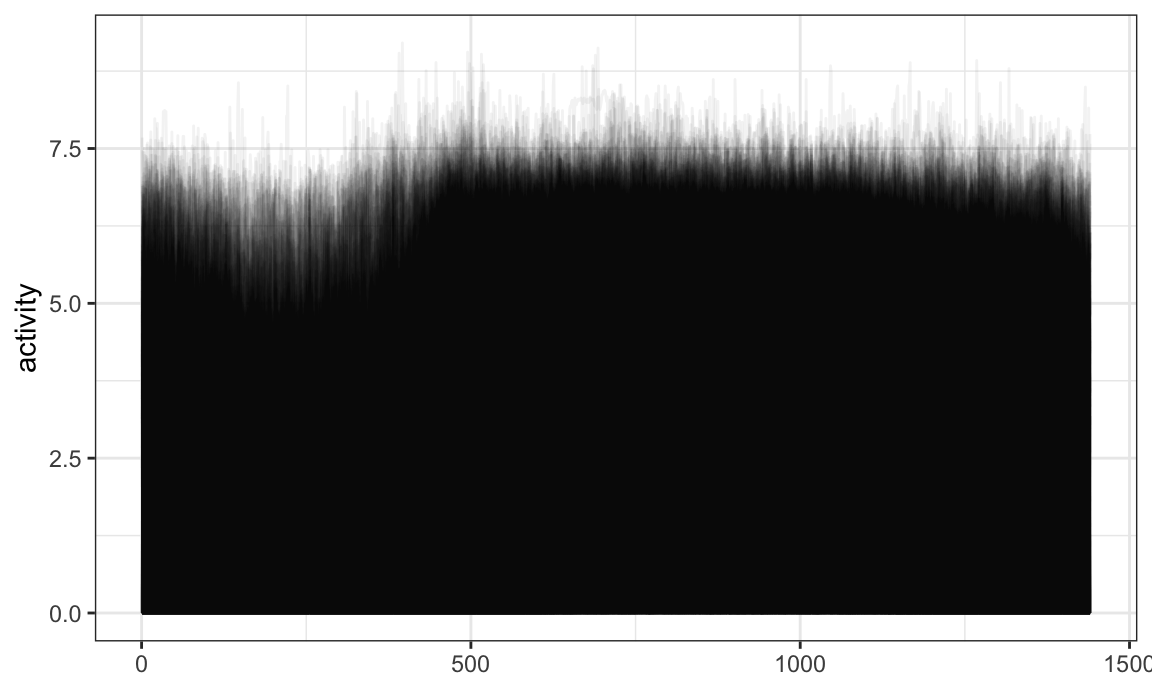The tidyfun::dti_df contains fractional anisotropy (FA) tract profiles for the corpus callosum (cca) and the right corticospinal tract (rcst), along with several covariates.

data(dti_df)

A quick plot of the cca tract profiles is below.

dti_df %>%
ggplot(aes(y = cca)) +
geom_spaghetti(alpha = .05)
## Warning: Unknown or uninitialised column: flipped_aes.

## Warning: Unknown or uninitialised column: flipped_aes.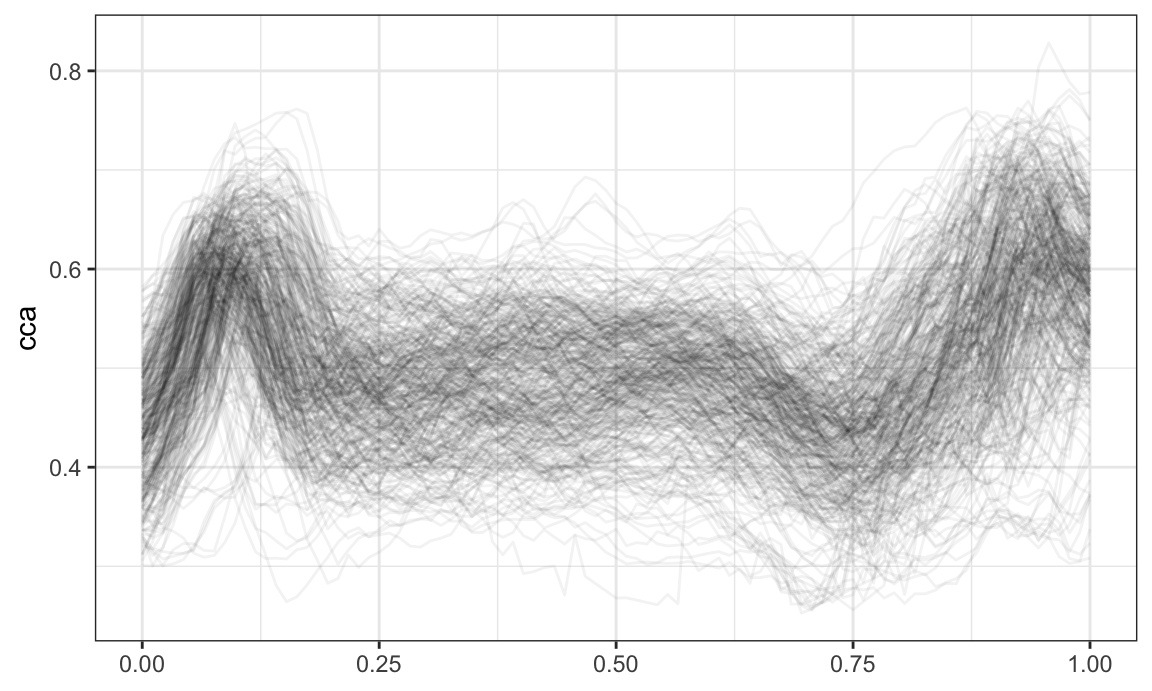### tf vectors

tf is a new data type for (vectors of) functional data. It contains subclasses for “raw” functional data (tfd) that can be dense / sparse and regular / irregular, and for “basis representation” functional data (tfb). Internally, there are attributes that define function-like behavior, including evaluation for new arguments, resolution, and the functional domain.

First I’ll pull a tf vector from the tidyfun::dti_df dataset. The resulting vector contain fractional anisotropy tract profiles for the corpus callosum (cca). When printed, tf vectors show the first few arg and value pairs for each subject.

data("dti_df")

cca_five = dti_df$cca[1:5] cca_five ## tfd on (0,1) based on 93 to 93 (mean: 93) evaluations each ## inter-/extrapolation by tf_approx_linear ## 1001_1: (0.000,0.49);(0.011,0.52);(0.022,0.54); ... ## 1002_1: (0.000,0.47);(0.011,0.49);(0.022,0.50); ... ## 1003_1: (0.000,0.50);(0.011,0.51);(0.022,0.54); ... ## 1004_1: (0.000,0.40);(0.011,0.42);(0.022,0.44); ... ## 1005_1: (0.000,0.40);(0.011,0.41);(0.022,0.40); ... For illustration, we plot the vector cca_five below. plot(cca_five)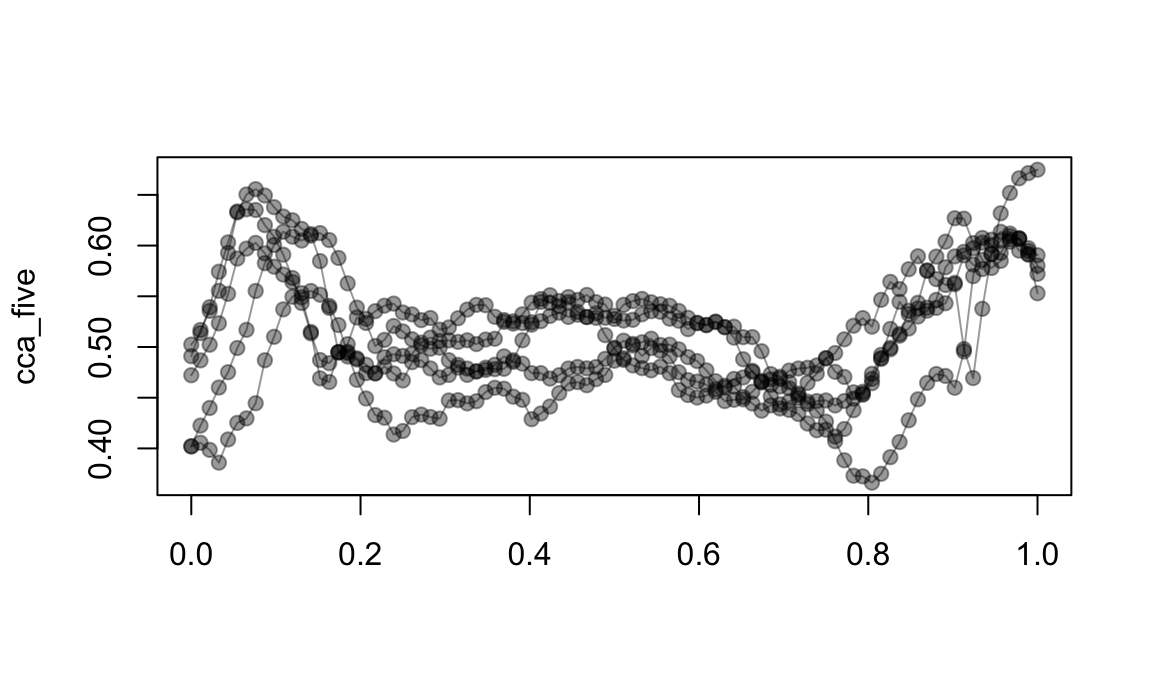Converting “raw” to “basis” representation is possible, and introduces some smoothing by default. cca_five_b = cca_five %>% tfb() ## Percentage of input data variability preserved in basis representation ## (per functional observation, approximate): ## Min. 1st Qu. Median Mean 3rd Qu. Max. ## 95.60 96.40 96.90 97.12 98.00 98.70 A quick plot illustrates the difference visually; lots of things are different in the background! plot(cca_five) lines(cca_five_b, col = "red")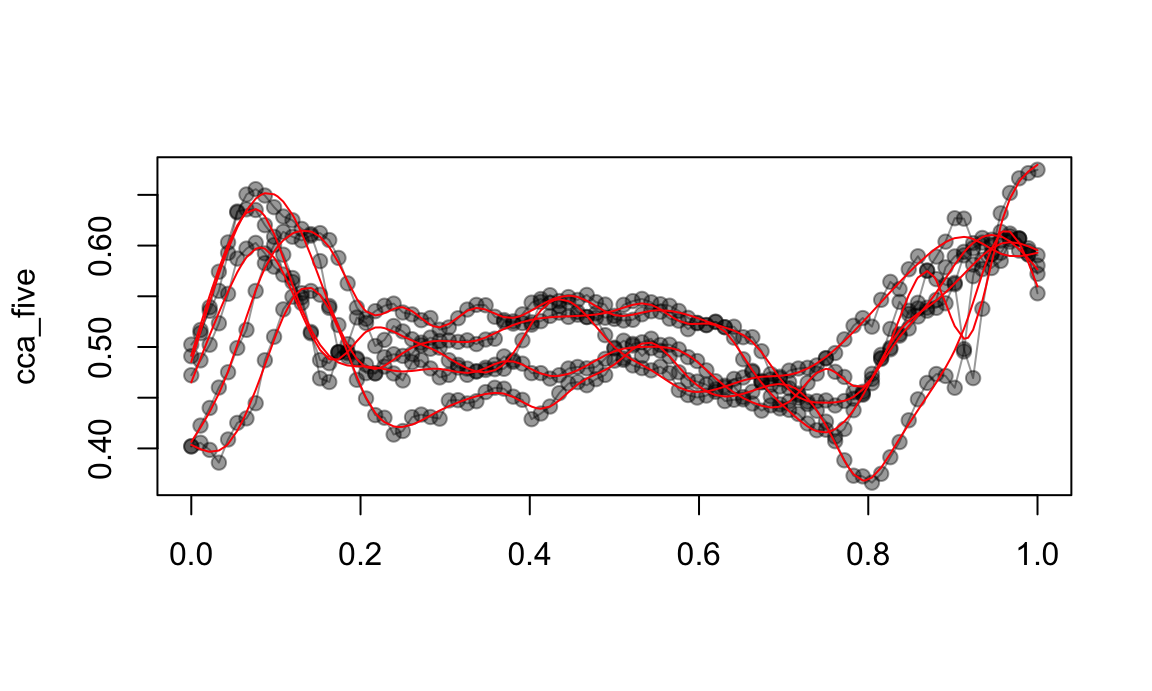### Operations on tf vectors The following is a brief overview of the kinds of operations available for tf vectors. You can perform basic arithmetic and logical comparisons: cca_five + cca_five == 2 * cca_five ## 1001_1 ## TRUE log(exp(cca_five)) == cca_five ## 1002_1 ## TRUE cca_five - (2:-2) != cca_five ## 1001_1 1002_1 1003_1 1004_1 1005_1 ## TRUE TRUE FALSE TRUE TRUE You can summarize using mean, sd, and other functions: c(mean = mean(cca_five), sd = sd(cca_five)) ## tfd on (0,1) based on 93 to 93 (mean: 93) evaluations each ## inter-/extrapolation by tf_approx_linear ## mean: (0.000, 0.45);(0.011, 0.47);(0.022, 0.48); ... ## sd: (0.000,0.049);(0.011,0.052);(0.022,0.062); ... You can determine whether a function satisfies a logical condition anywhere: cca_five %>% tf_anywhere(value > .65) ## 1001_1 1002_1 1003_1 1004_1 1005_1 ## TRUE FALSE TRUE FALSE FALSE And you can zoom in on regions of interest: cca_five_zoom = cca_five %>% tf_zoom(.5, 1) plot(cca_five_zoom)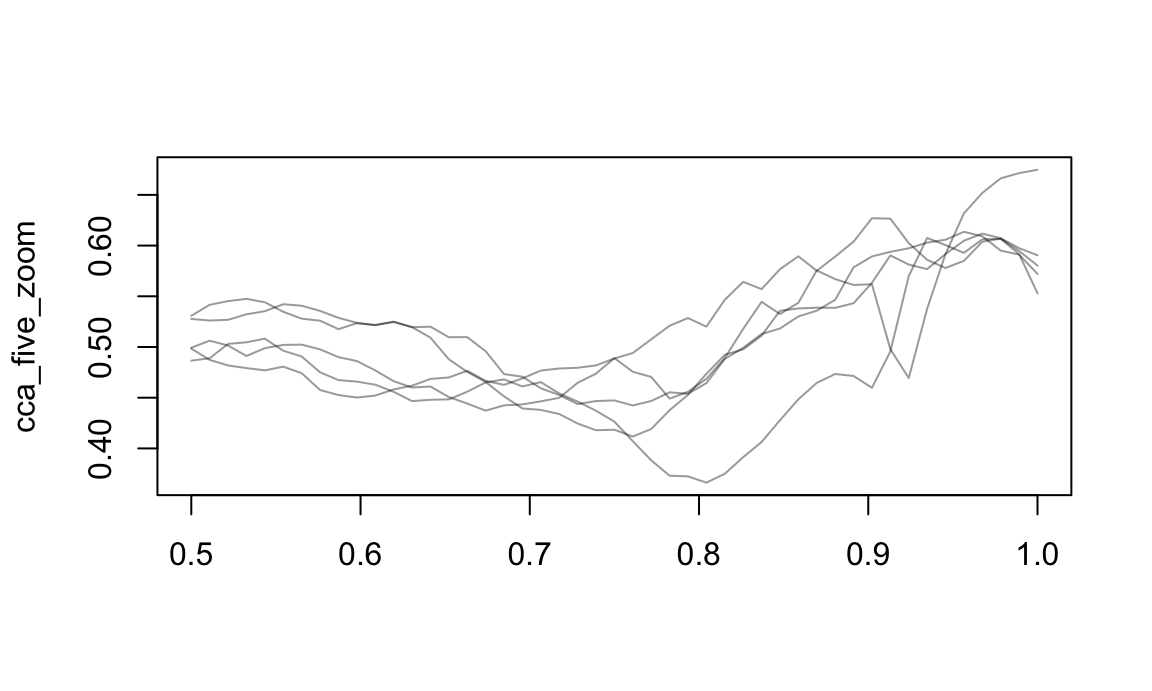### tf vectors in dataframes The main goal of tidyfun is to ease exploratory analysis by putting functional data in data frames. Since tf vectors are treated the same way as vectors of class numeric or factor, they can enter dataframes the same way. The DTI data, for example, include scalar covariates and two functional variables: dti_df ## # A tibble: 382 x 6 ## id visit sex case cca ## <dbl> <int> <fct> <fct> <tfd_irrg> ## 1 1001 1 fema… cont… 1001_1: (0.000,0.49);(0.011,0.52);(0.022,0.54); ... ## 2 1002 1 fema… cont… 1002_1: (0.000,0.47);(0.011,0.49);(0.022,0.50); ... ## 3 1003 1 male cont… 1003_1: (0.000,0.50);(0.011,0.51);(0.022,0.54); ... ## 4 1004 1 male cont… 1004_1: (0.000,0.40);(0.011,0.42);(0.022,0.44); ... ## 5 1005 1 male cont… 1005_1: (0.000,0.40);(0.011,0.41);(0.022,0.40); ... ## 6 1006 1 male cont… 1006_1: (0.000,0.45);(0.011,0.45);(0.022,0.46); ... ## 7 1007 1 male cont… 1007_1: (0.000,0.55);(0.011,0.56);(0.022,0.56); ... ## 8 1008 1 male cont… 1008_1: (0.000,0.45);(0.011,0.48);(0.022,0.50); ... ## 9 1009 1 male cont… 1009_1: (0.000,0.50);(0.011,0.51);(0.022,0.52); ... ## 10 1010 1 male cont… 1010_1: (0.000,0.46);(0.011,0.47);(0.022,0.48); ... ## # … with 372 more rows, and 1 more variable: rcst <tfd_irrg> And the CHF data is an example of a multilevel dataset with a functional observation: chf_df ## # A tibble: 329 x 8 ## id gender age bmi event_week event_type day ## <dbl> <chr> <dbl> <dbl> <dbl> <chr> <ord> ## 1 1 Male 41 26 41 . Mon ## 2 1 Male 41 26 41 . Tue ## 3 1 Male 41 26 41 . Wed ## 4 1 Male 41 26 41 . Thu ## 5 1 Male 41 26 41 . Fri ## 6 1 Male 41 26 41 . Sat ## 7 1 Male 41 26 41 . Sun ## 8 3 Female 81 21 32 . Mon ## 9 3 Female 81 21 32 . Tue ## 10 3 Female 81 21 32 . Wed ## # … with 319 more rows, and 1 more variable: activity <tfd_reg> Now our functional data are tidy! That is, we have data rectangles, and each functional observation exists in a single “cell”. ### Data wrangling Dataframes using tidyfun to store functional observations can be manipulated using tools from dplyr, including select and filter: chf_df %>% select(id, day, activity) %>% filter(day == "Mon") %>% ggplot(aes(y = activity)) + geom_spaghetti(alpha = .05) ## Warning: Unknown or uninitialised column: flipped_aes. ## Warning: Unknown or uninitialised column: flipped_aes.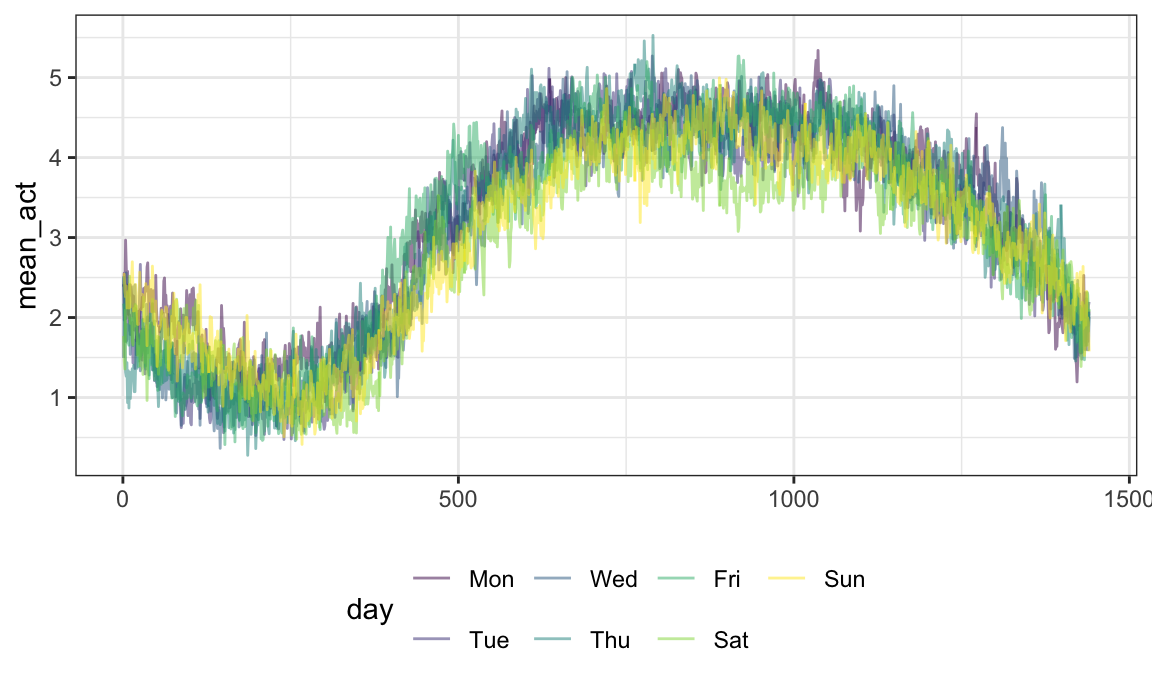Operations using group_by and summarize are allowed: chf_df %>% group_by(day) %>% summarize(mean_act = mean(activity)) %>% ggplot(aes(y = mean_act, color = day)) + geom_spaghetti() ## summarise() ungrouping output (override with .groups argument) ## Warning: Unknown or uninitialised column: flipped_aes. ## Warning: Unknown or uninitialised column: flipped_aes.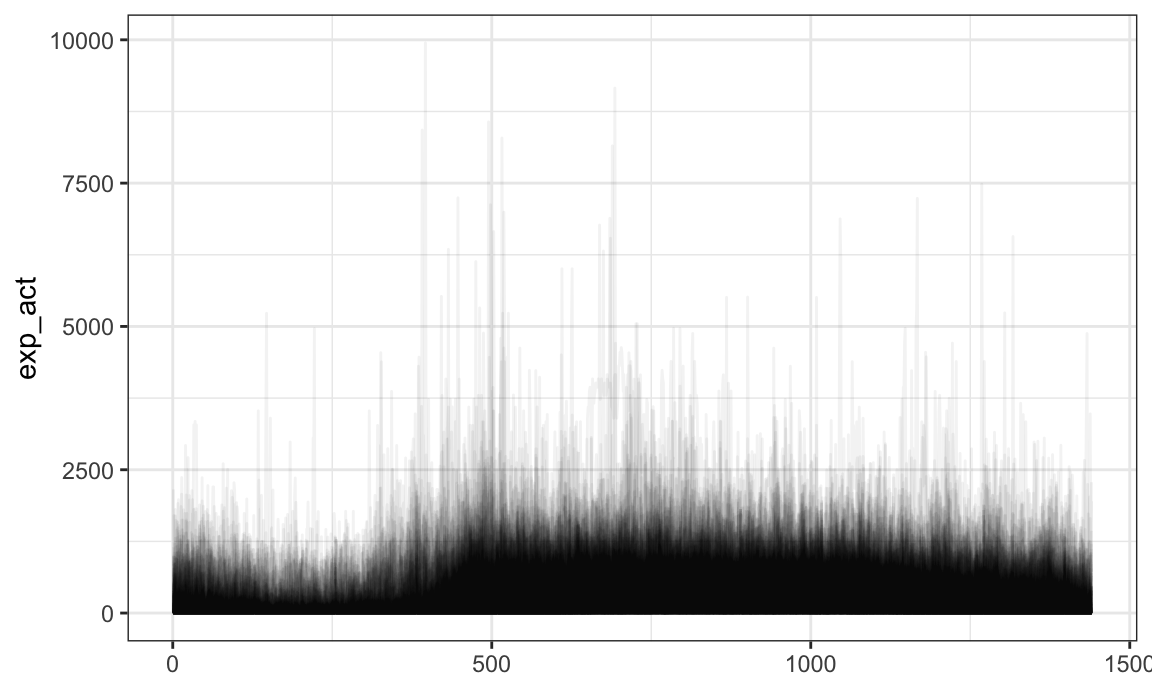One can mutate functional observations – here we exponentiate the log activity counts to obtain original recordings: chf_df %>% mutate(exp_act = exp(activity)) %>% ggplot(aes(y = exp_act)) + geom_spaghetti(alpha = .05) ## Warning: Unknown or uninitialised column: flipped_aes. ## Warning: Unknown or uninitialised column: flipped_aes.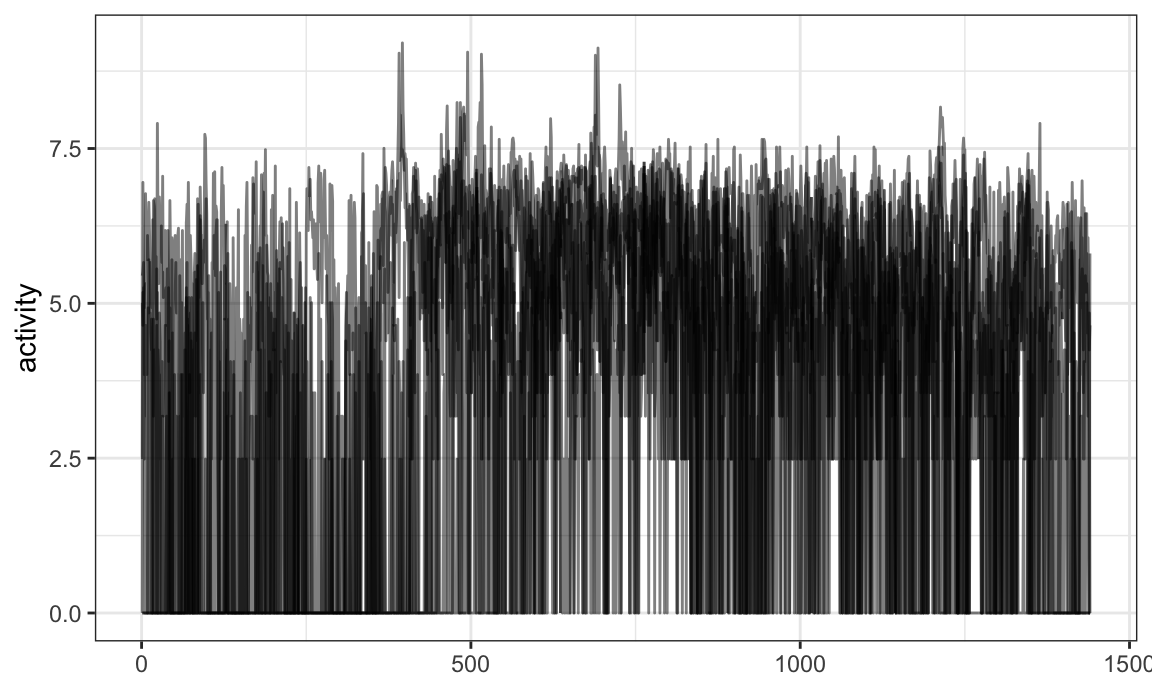Some dplyr functions are most useful in conjunction with new functions in tidyfun. For example, one might use filter with tf_anywhere to filter based on the values of observed functions: chf_df %>% filter(tf_anywhere(activity, value > 9)) %>% ggplot(aes(y = activity)) + geom_spaghetti() ## Warning: Unknown or uninitialised column: flipped_aes. ## Warning: Unknown or uninitialised column: flipped_aes.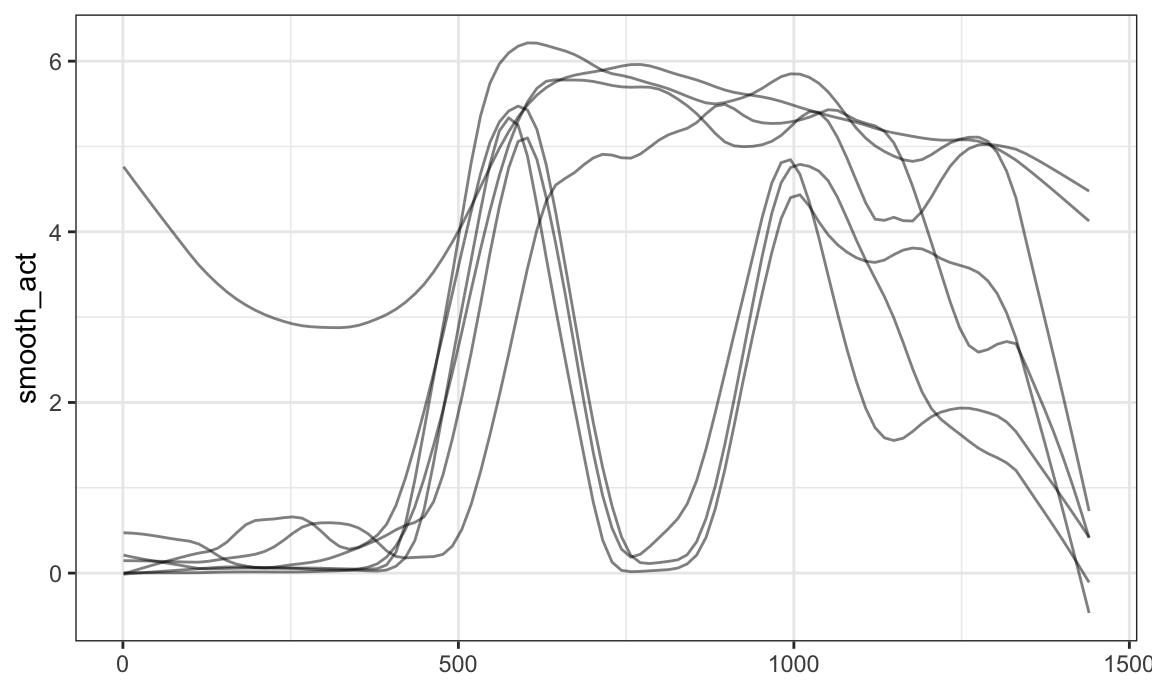One can add smoothed versions of existing observations using mutate and tf_smooth: chf_df %>% filter(id == 1) %>% mutate(smooth_act = tf_smooth(activity)) %>% ggplot(aes(y = smooth_act)) + geom_spaghetti() ## using f = 0.15 as smoother span for lowess ## Warning: Unknown or uninitialised column: flipped_aes. ## Warning: Unknown or uninitialised column: flipped_aes.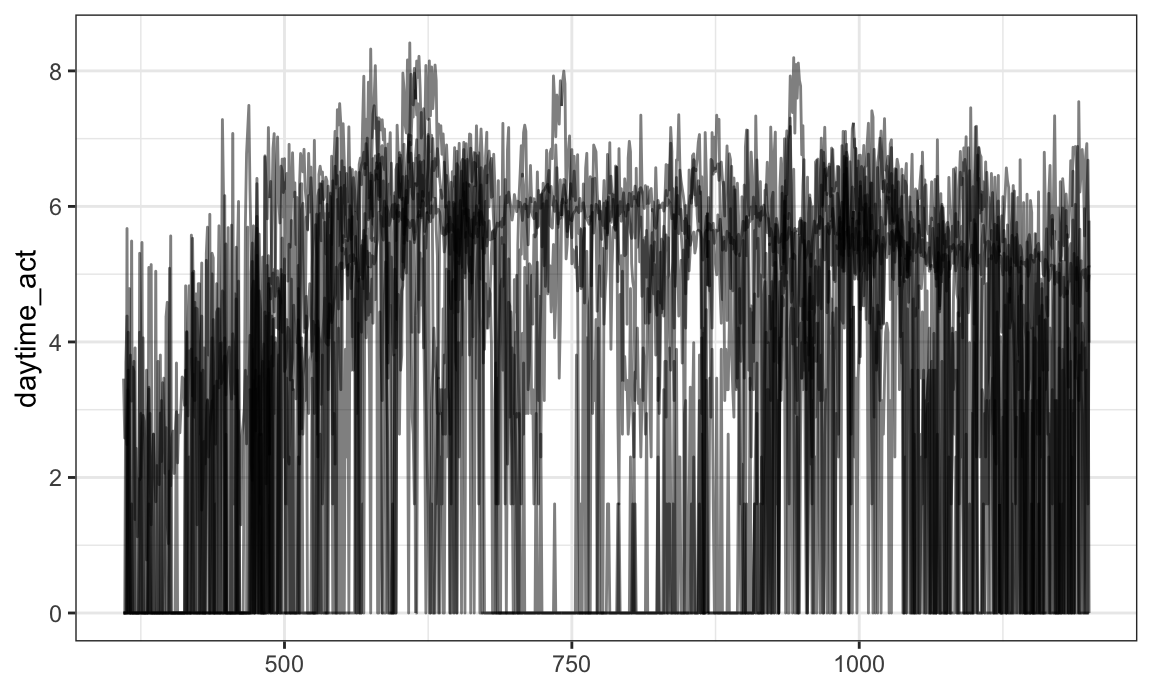One can also extract observations over a subset of the full domain using mutate and tf_zoom: chf_df %>% filter(id == 1) %>% mutate(daytime_act = tf_zoom(activity, 360, 1200)) %>% ggplot(aes(y = daytime_act)) + geom_spaghetti() ## Warning: Unknown or uninitialised column: flipped_aes. ## Warning: Unknown or uninitialised column: flipped_aes.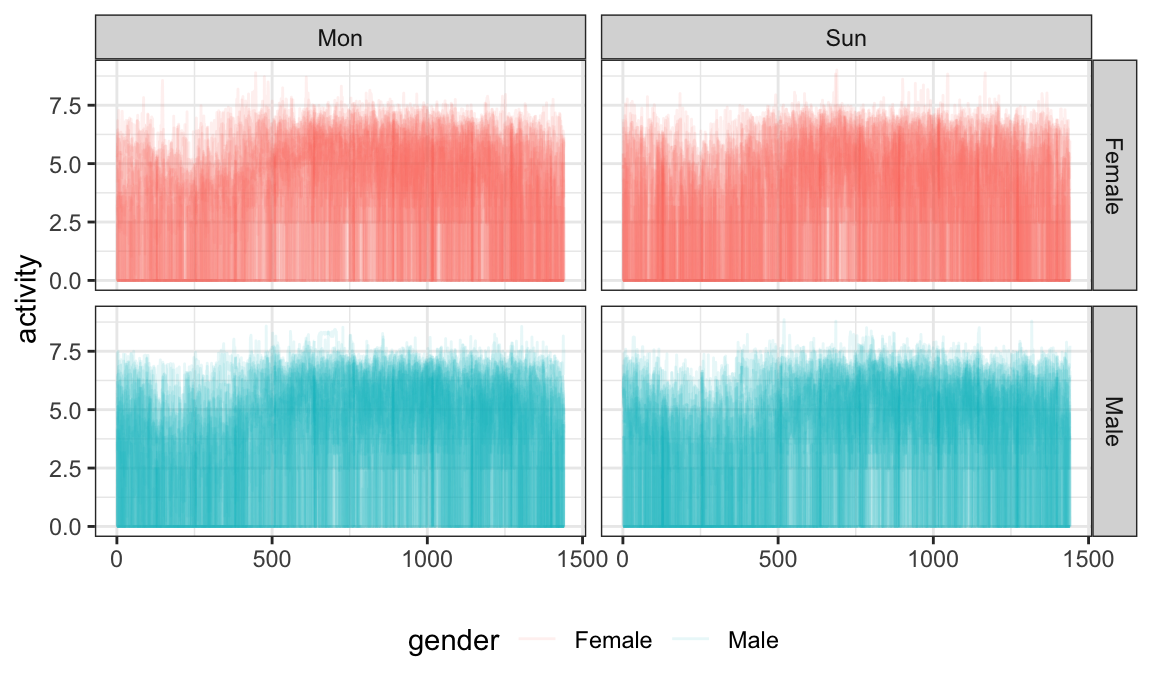In general, EDA for functional data using tidyverse tools is now possible, and is often most powerful when paired with new functions in tidyfun. ### Visualization We’ve seen both plot and geom_spaghetti to aid in understanding some content to this point – base R for tf vectors, ggplot for tidy data. You can use more advanced options and combine with data wrangling steps. You can use facetting: chf_df %>% filter(day %in% c("Mon", "Sun")) %>% ggplot(aes(y = activity, color = gender)) + geom_spaghetti(alpha = .1) + facet_grid(gender ~ day) ## Warning: Unknown or uninitialised column: flipped_aes. ## Warning: Unknown or uninitialised column: flipped_aes.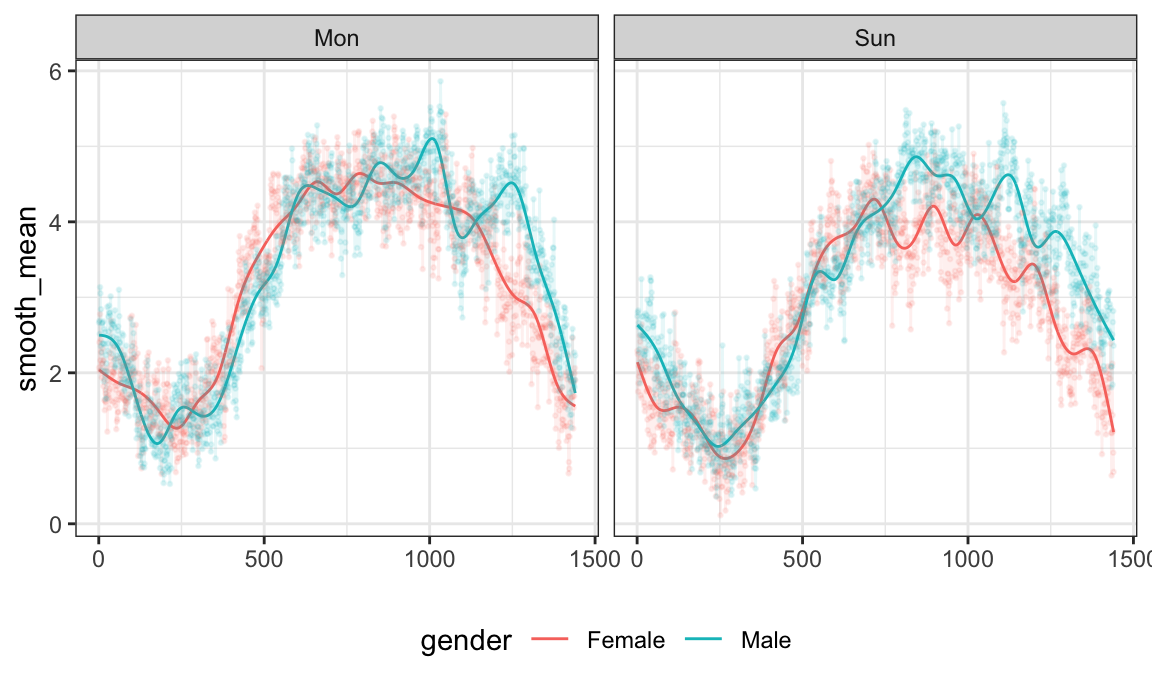Together with data manipulation tools, the integration with ggplot can produce useful exploratory analyses. Note that this plot also introduces geom_meatballs(): chf_df %>% group_by(gender, day) %>% summarize(mean_act = mean(activity)) %>% mutate(smooth_mean = tfb(mean_act)) %>% filter(day %in% c("Mon", "Sun")) %>% ggplot(aes(y = smooth_mean, color = gender)) + geom_spaghetti(size = 1.25, alpha = 1) + geom_meatballs(aes(y = mean_act), alpha = .1) + facet_grid(~ day) ## summarise() regrouping output by 'gender' (override with .groups argument) ## Percentage of input data variability preserved in basis representation ## (per functional observation, approximate): ## Min. 1st Qu. Median Mean 3rd Qu. Max. ## 88.70 91.35 92.00 91.56 92.25 93.00 ## Percentage of input data variability preserved in basis representation ## (per functional observation, approximate): ## Min. 1st Qu. Median Mean 3rd Qu. Max. ## 89.00 91.80 93.00 92.14 93.05 93.30 ## Percentage of input data variability preserved in basis representation ## (per functional observation, approximate): ## Min. 1st Qu. Median Mean 3rd Qu. Max. ## 100 100 100 100 100 100 ## Percentage of input data variability preserved in basis representation ## (per functional observation, approximate): ## Min. 1st Qu. Median Mean 3rd Qu. Max. ## 100 100 100 100 100 100 ## Warning: Unknown or uninitialised column: flipped_aes. ## Warning: Unknown or uninitialised column: flipped_aes. ## Warning: Unknown or uninitialised column: flipped_aes. ## Warning: Unknown or uninitialised column: flipped_aes.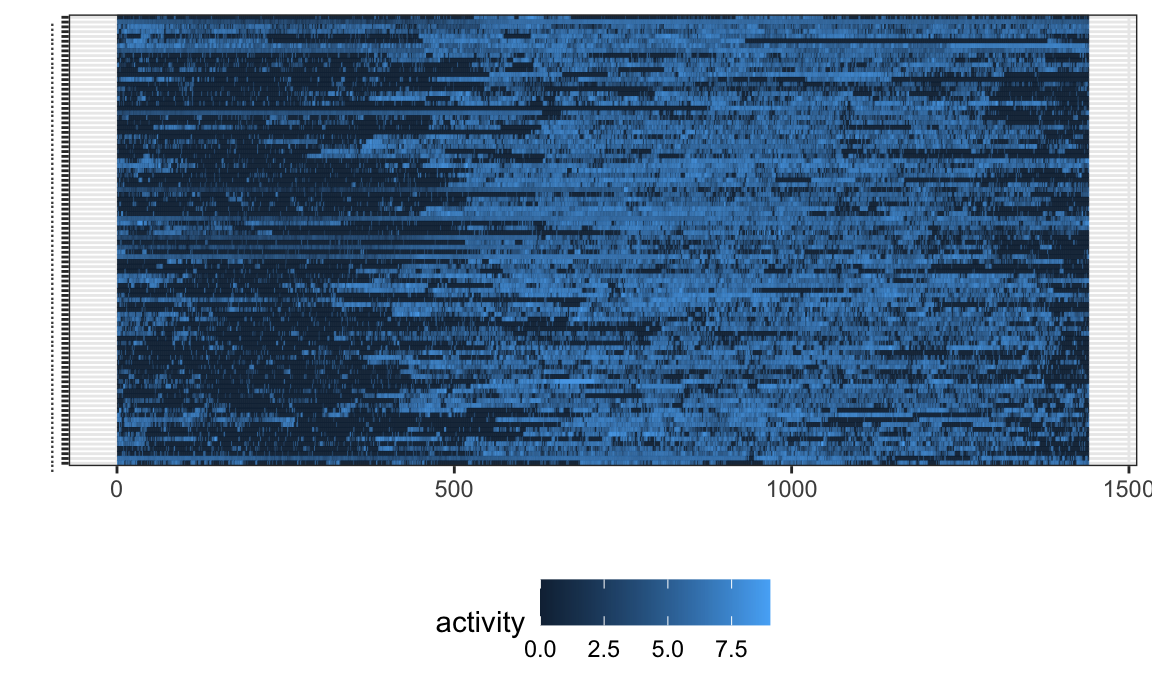Lasagna plots are a variant on a heatmaps which show functional observations in rows and use color to illustrate values taken at different arguments. In tidyfun, lasagna plots are implemented through gglasagna. A first example, using the CHF data, is below. chf_df %>% filter(day %in% c("Mon", "Sun")) %>% gglasagna(activity)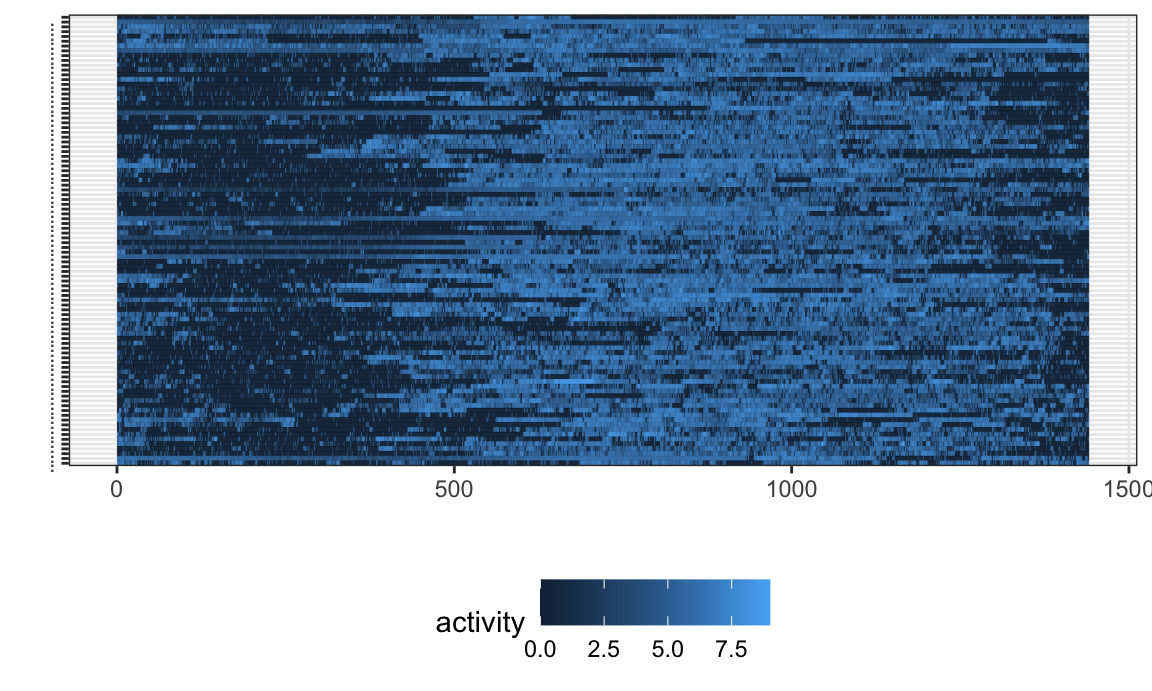A somewhat more involved example, demonstrating the order argument and taking advantage of facetting, is next. dti_df %>% gglasagna( y = cca, order = tf_integrate(cca, definite = TRUE), arg = seq(0,1, l = 101)) + theme(axis.text.y = element_text(size = 6)) + facet_wrap(~ case, ncol = 2, scales = "free")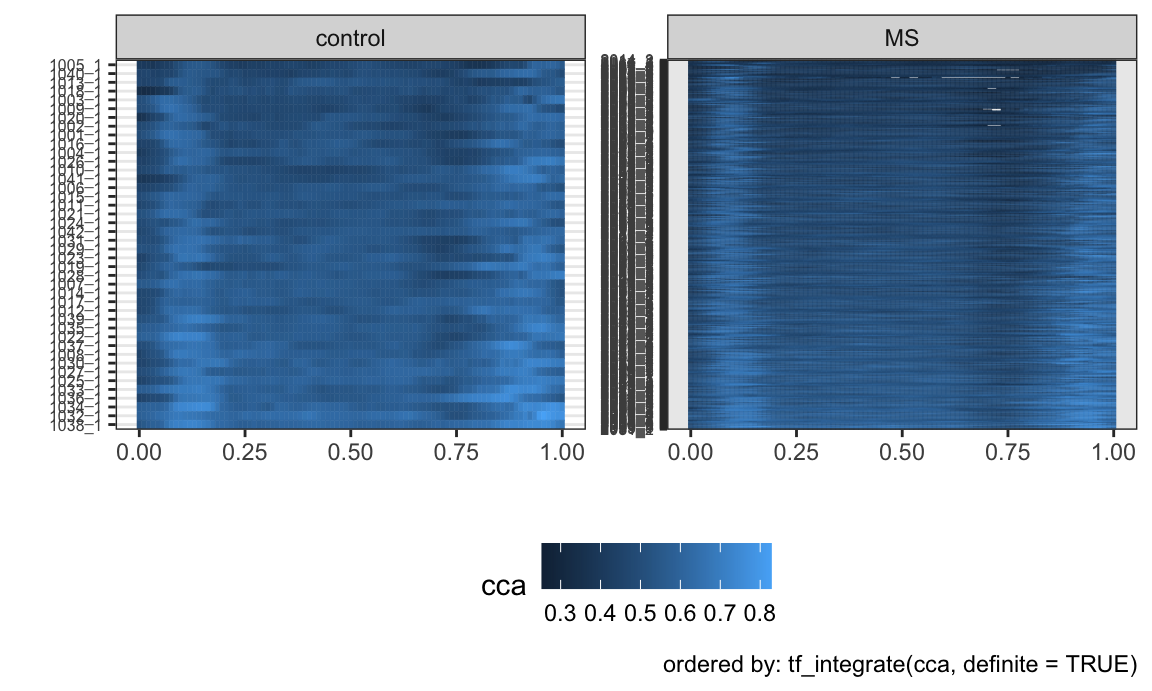### Conversion The DTI data in the refund package has been a popular example in functional data analysis. In the code below, we create a data frame (or tibble) containing scalar covariates, and then add columns for the cca and rcst track profiles. This code was used to create the tidyfun::dti_df dataset included in the package. dti_df = tibble( id = refund::DTI$ID,
sex = refund::DTI$sex, case = factor(ifelse(refund::DTI$case, "MS", "control")))

dti_df$cca = tfd(refund::DTI$cca, arg = seq(0,1, l = 93))
dti_df$rcst = tfd(refund::DTI$rcst, arg = seq(0, 1, l = 55))

“Long” format data frames containing functional data include columns containing a subject identifier, the functional argument, and the value each subject’s function takes at each argument. There are also often (but not always) non-functional covariates that are repeated within a subject. For data in this form, we use tf_nest to produce a data frame containing a single row for each subject.

An example is the pig weight data from the SemiPar package, which is a nice example from longitudinal data analysis. This includes columns for id.num, num.weeks, and weight – which correspond to the subject, argument, and value.

data("pig.weights", package = "SemiPar")

pig.weights = as_tibble(pig.weights)

pig.weights
## # A tibble: 432 x 3
##    id.num num.weeks weight
##     <int>     <int>  <dbl>
##  1      1         1   24
##  2      1         2   32
##  3      1         3   39
##  4      1         4   42.5
##  5      1         5   48
##  6      1         6   54.5
##  7      1         7   61
##  8      1         8   65
##  9      1         9   72
## 10      2         1   22.5
## # … with 422 more rows

We create pig_df by nesting weight within subject. The result is a data frame containing a single row for each pig, and columns for id.num and the weight function.

pig_df =
pig.weights %>%
tf_nest(weight, .id = id.num, .arg = num.weeks)

pig_df
## # A tibble: 48 x 2
##    id.num                        weight
##     <int>                     <tfd_reg>
##  1      1  1: (1,24);(2,32);(3,39); ...
##  2      2  2: (1,22);(2,30);(3,40); ...
##  3      3  3: (1,22);(2,28);(3,36); ...
##  4      4  4: (1,24);(2,32);(3,40); ...
##  5      5  5: (1,24);(2,32);(3,37); ...
##  6      6  6: (1,23);(2,30);(3,36); ...
##  7      7  7: (1,22);(2,28);(3,36); ...
##  8      8  8: (1,24);(2,30);(3,38); ...
##  9      9  9: (1,20);(2,28);(3,33); ...
## 10     10 10: (1,26);(2,32);(3,40); ...
## # … with 38 more rows

Additional functions allow conversion from other data structures to tf vectors, and also implement conversions back to these data structures.

## Some FPCA

# irregular data
data(dti_df)
dti_fpca = rfr_fpca(Y = "cca", data = dti_df)

dti_df %>%
filter(case == "control") %>%
ggplot(aes(y = cca_fits)) +
geom_spaghetti(alpha = .4) +
geom_spaghetti(aes(y = cca), color = "blue", alpha = .3) +
facet_grid(~sex)
## Warning: Unknown or uninitialised column: flipped_aes.

## Warning: Unknown or uninitialised column: flipped_aes.

## Warning: Unknown or uninitialised column: flipped_aes.

## Warning: Unknown or uninitialised column: flipped_aes.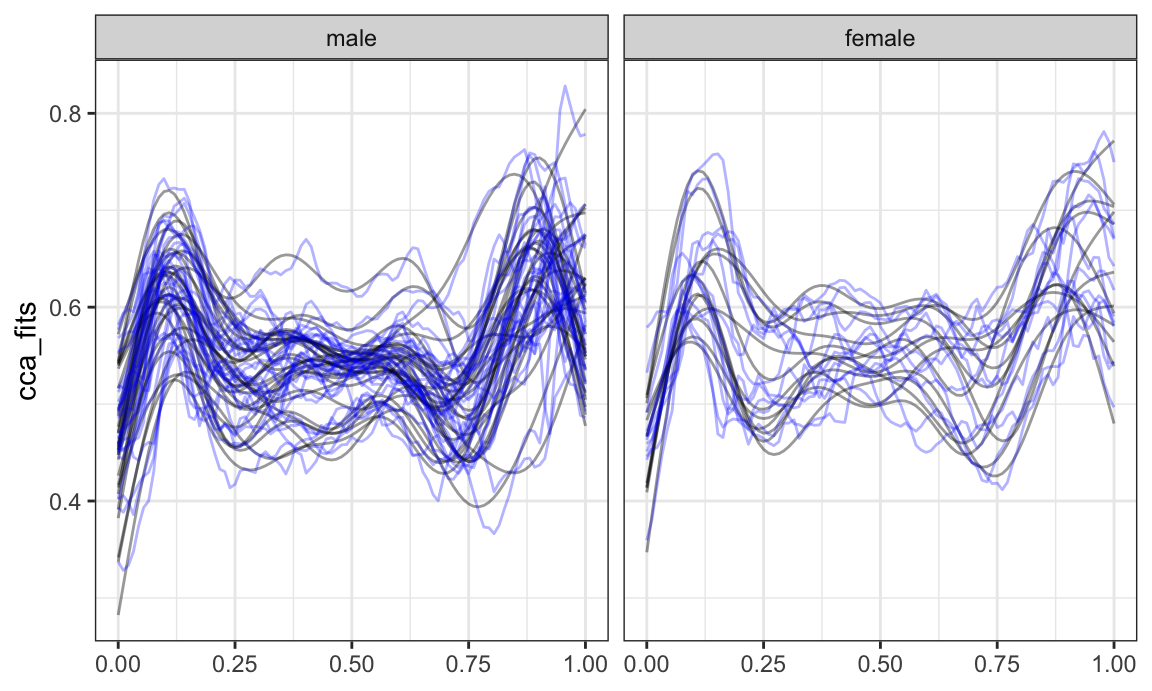## Next steps

tidyfun itself is a work in progress:

• There are several open issues / to dos / bug fixes
• We have some ideas for new features
• User feedback will help!

Integration with analysis is longer-term goal:

• Some refund functions have been unofficially updated to work with dataframe / tf intpus
• More robust / complete integration is needed